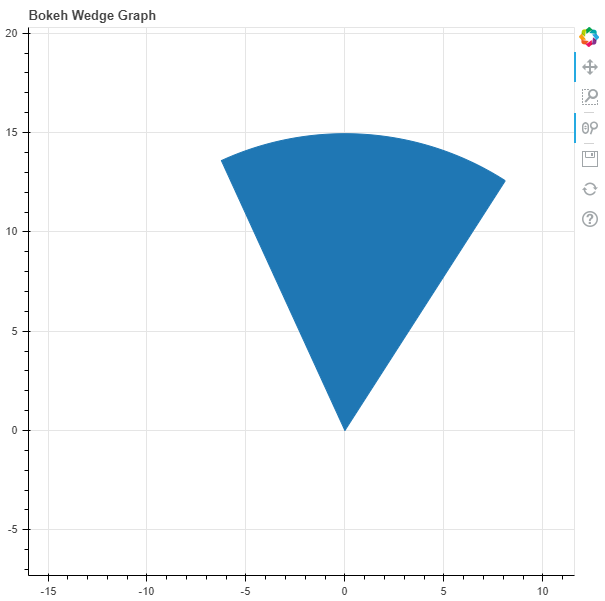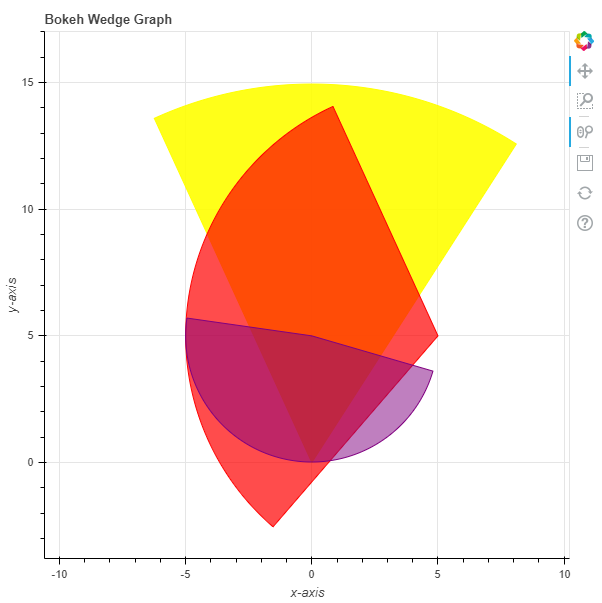Open in App
Not now

# Python Bokeh – Plotting Wedges on a Graph

• Last Updated : 10 Jul, 2020

Bokeh is a Python interactive data visualization. It renders its plots using HTML and JavaScript. It targets modern web browsers for presentation providing elegant, concise construction of novel graphics with high-performance interactivity.

Bokeh can be used to plot wedges on a graph. Plotting wedges on a graph can be done using the `wedge()` method of the `plotting` module.

## plotting.figure.wedge()

Syntax : wedge(parameters)

Parameters :

• x : x-coordinates of the wedge markers
• y : y-coordinates of the wedge markers
• start_angle : angle to start the wedge markers
• start_angle_units : unit of the angle to start the wedge markers, default is radian
• end_angle : angle to end the wedge markers
• end_angle_units : unit of the angle to end the wedge markers
• direction : direction of strokes, default is anticlock

Returns : an object of class `GlyphRenderer`

Example 1 : In this example we will be using the default values for plotting the graph.

 `# importing the modules``from` `bokeh.plotting ``import` `figure, output_file, show``        ` `# file to save the model``output_file(``"gfg.html"``)``        ` `# instantiating the figure object``graph ``=` `figure(title ``=` `"Bokeh Wedge Graph"``)``      ` `# the points to be plotted``x ``=` `0``y ``=` `0`` ` `# radius of the wedge``radius ``=` `15`` ` `# start angle of the wedge``start_angle ``=` `1`` ` `# end angle of the wedge``end_angle ``=` `2`` ` `# plotting the graph``graph.wedge(x, y,``            ``radius ``=` `radius,``            ``start_angle ``=` `start_angle,``            ``end_angle ``=` `end_angle)``      ` `# displaying the model``show(graph)`

Output :Example 2 : In this example we will be plotting multiple wedges with various other parameters.

 `# importing the modules``from` `bokeh.plotting ``import` `figure, output_file, show``        ` `# file to save the model``output_file(``"gfg.html"``)``        ` `# instantiating the figure object``graph ``=` `figure(title ``=` `"Bokeh Wedge Graph"``)`` ` `# name of the x-axis ``graph.xaxis.axis_label ``=` `"x-axis"``       ` `# name of the y-axis ``graph.yaxis.axis_label ``=` `"y-axis"`` ` `# the points to be plotted``x ``=` `[``0``, ``5``, ``0``]``y ``=` `[``0``, ``5``, ``5``]`` ` `# radius of the wedges``radius ``=` `[``15``, ``10``, ``5``]`` ` `# start angle of the wedges``start_angle ``=` `[``1``, ``2``, ``3``]`` ` `# end angle of the wedges``end_angle ``=` `[``2``, ``4``, ``6``]`` ` `# color value of the wedges``color ``=` `[``"yellow"``, ``"red"``, ``"purple"``]``  ` `# fill alpha value of the wedges``fill_alpha ``=` `[``0.9``, ``0.7``, ``0.5``]`` ` `# plotting the graph``graph.wedge(x, y,``            ``radius ``=` `radius,``            ``start_angle ``=` `start_angle,``            ``end_angle ``=` `end_angle,``            ``color ``=` `color,``            ``fill_alpha ``=` `fill_alpha)``      ` `# displaying the model``show(graph)`

Output :My Personal Notes arrow_drop_up